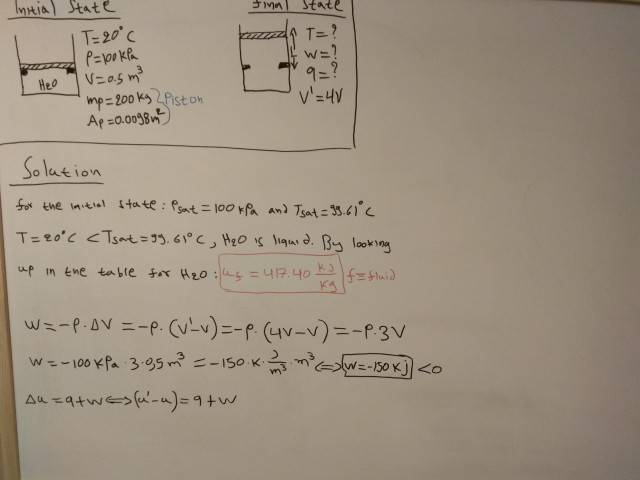# Thermodynamics simple problem.

babaliaris
Moved from technical forums, so no template
I'm new to thermodynamics and after some reading I tried to solve the problem below but I have stuck (I think this problem assumes you know only the first law of thermodynamics)You have a piston resting on some stops inside a container filled with water and you want to find out after heating the container the final Temperature(T), the Work(w) that has been done and the Heat(q) that was transferred. The final state has 4 times more volume than the initial state.

I found the work with pressure constant by using the formula
w = -P ⋅ ΔV

but I don't know how to find the heat. I wrote down the first law
Δu = q+w

Also notice that in the above formula all quantities are per mass for some reason so the work which I calculated before probably needs to be divided by the pistons mass or something before I use it in this formula???

but I only know the work and the initial internal energy, I don't know the final internal energy so I can't find Δu => I can't find q.

Is there something that I'm missing? I used tables to find the internal energy (u) in the initial state (Notice in the picture that u (in red) is kj/kg).

## Answers and Replies

Christopher Grayce
How is the pressure constant? The piston starts off resting on stops, and unless it is barely resting there, that means P inside the gas is less than when the piston is being supported by the gas, after the expansion. You might try calculating the work by m*g*dh, where m = mass of piston, g = 9.8 m/s^2, and dh is distance piston moves, which you can get by using the area of the piston and volume of the cylinder to work out the diameter of the cylinder, then using this with the change in volume to calculate dh.

babaliaris
How is the pressure constant? The piston starts off resting on stops, and unless it is barely resting there, that means P inside the gas is less than when the piston is being supported by the gas, after the expansion. You might try calculating the work by m*g*dh, where m = mass of piston, g = 9.8 m/s^2, and dh is distance piston moves, which you can get by using the area of the piston and volume of the cylinder to work out the diameter of the cylinder, then using this with the change in volume to calculate dh.

OK I did not know that. So I will use force times distance to calculate work (makes sense).
1) I don't really know how to calculate dh using what you said.
2) Why work = m⋅g⋅dh ? A force must push the piston to go up so weight should not be the only force.

Now something else. If I was to say from 1 to 10 what is my current knowledge of physics I would say about 3.
How much will this affect me from learning Thermodynamics?

Until know these are what I know from physics:
1) Movements
2) Newton's Laws.
3) Work

Christopher Grayce
1. The notes you show in your post contain the area of the piston, which corresponds to the area of the cylinder. You know the initial and final volumes of the cylinder, so you use the formula for the volume of a cylinder (V = A*h) and the area of the piston to get the initial and final heights of the piston.

2. The only force pushing down on the piston is its weight, F = m*g, where m = mass of piston and g = acceleration due to gravity, 9.8 m/s^2. When the piston is pushed up the force from the gas pushing up will be approximately equal to the weight of the piston pushing down. (If it were less, the piston wouldn't move at all, and if it were significantly more the piston would move abruptly and chaotically, and normally we assume that doesn't happen in thermo, because that kind of change is difficult to analyze.) Work is force times distance, so F = m*g times the distance dh = h_final - h_initial the piston moves, so that's where I get m*g*dh.

3. I don't know. Thermodynamics depends somewhat on an understanding of mechanics (forces, energy), but not hugely. It depends a lot on what the focus of your thermodynamics is (e.g. engineering, chemistry, biology), and on how you're learning it (e.g. on your own or in a class). If you're learning it in a class, my advice would be to talk to the instructor. Even if his assumptions about how much physics you know doesn't match reality, he will have suggestions (certainly better than mine) about how to supplement what you already know efficiently.

•babaliaris
Mentor
Let's assume that there is vacuum outside the cylinder and piston. From a force balance on the piston, what water vapor pressure is required to just begin levitating the piston? From the saturated steam tables, what temperature does this correspond to? What is the mass of water within the cylinder? What is the initial specific internal energy of the water within the cylinder? What is the initial internal energy of the mass of water within the cylinder? What is the specific internal energy of the water when the piston just begins to levitate? What is the internal energy of the mass of water when the piston just begins to levitate? How much work has been done at this point? What is the change in internal energy up to the point that the piston just begins levitating? How much heat is transferred up to this point?

Mentor
Is it possible that they mean that the pressure outside the cylinder is 100 kPa?